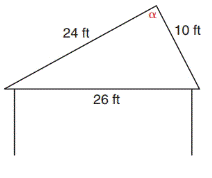Chapter 11.4, Problem 32E### Elementary Geometry for College St...

6th Edition
Daniel C. Alexander + 1 other
ISBN: 9781285195698

#### Solutions

Chapter
Section### Elementary Geometry for College St...

6th Edition
Daniel C. Alexander + 1 other
ISBN: 9781285195698
Textbook Problem
1 views

# In Exercises 29 to 34, use the Law of Sines or the Law of Cosines to solve each problem. Angle measures should be found to the nearest degree and areas and distances to the nearest tenth of a unit.Above one room of a house the rafters meet as shown. What is the measure of the angle α at which they meet?To determine

To find:

The measure of the angle α.

Explanation

Formula:

The Law of Cosines

cosα=b2+c2a22bc

cosβ=a2+c2b22ac

cosγ=a2+b2c22ab

Where α, β, and γ is the acute angle of the triangle, and a, b, and c is the sides of the triangle.

Calculation:

Given,

From the figure,

a=26 ft

b=24 ft

c=10 ft

Find α:

The alternative from of the Law of Cosines,

cosα=b2+c2a22bc

### Still sussing out bartleby?

Check out a sample textbook solution.

See a sample solution

#### The Solution to Your Study Problems

Bartleby provides explanations to thousands of textbook problems written by our experts, many with advanced degrees!

Get Started